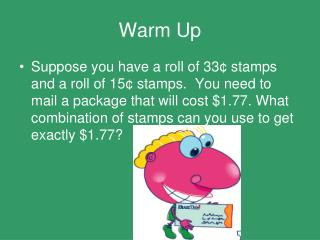DownloadDownload PresentationWarm Up

# Warm Up

Download Presentation## Warm Up

- - - - - - - - - - - - - - - - - - - - - - - - - - - E N D - - - - - - - - - - - - - - - - - - - - - - - - - - -
##### Presentation Transcript

1. Warm Up • Suppose you have a roll of 33¢ stamps and a roll of 15¢ stamps. You need to mail a package that will cost \$1.77. What combination of stamps can you use to get exactly \$1.77?

2. Solution • Four 33¢ stamps and three 15¢ stamps equals \$1.77 in postage.

3. Activity Time

4. Area of Parallelograms Lesson 11-4

5. Area of Parallelograms • In the Activity we found that the area of a parallelogram is similar to the area of a rectangle. • The area of a rectangle is l x w

6. Find the area of a parallelogram A = bh h b Remember the units when finding area of any figure are always SQUARED!! Parallelogram

7. Find the area of the figure round to the nearest tenth if necessary. • A = bh • A = 15cm x 12cm • A = 180 cm ² 12cm 15cm

8. Find the area of the figure round to the nearest tenth if necessary. 1.5m 0.75m • A = bh • A = 1.5m x 0.75m • A = 1.1 m ²

9. Find the area of the figure round to the nearest tenth if necessary. 2yd 3 ½ yd • A = bh • A = 3 ½ yd x 2 yd • A = 7 yd²

10. Find the area of the figure round to the nearest tenth if necessary. 7.2cm 7.9cm • A = bh • A = 7.2cm x 7.9cm • A = 56.9 cm ²

11. Don’t Forget • Homework: Lesson 11-4 # 1-14 all • Quiz: Next Tuesday on Lessons 11-3 Pythagorean Theorem and 11-4 Area of Parallelograms